Download Presentation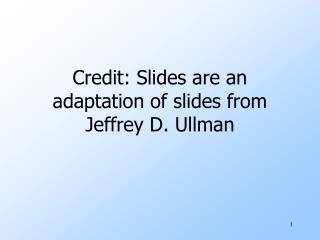Credit: Slides are an adaptation of slides from Jeffrey D. Ullman

# Credit: Slides are an adaptation of slides from Jeffrey D. Ullman - PowerPoint PPT Presentation

Credit: Slides are an adaptation of slides from Jeffrey D. Ullman. Conjunctive Queries. Containment Mappings Canonical Databases. Conjunctive Queries. A CQ is a single Datalog rule, with all subgoals assumed to be EDB.I am the owner, or an agent authorized to act on behalf of the owner, of the copyrighted work described.
Download Presentation## Credit: Slides are an adaptation of slides from Jeffrey D. Ullman

An Image/Link below is provided (as is) to download presentation

Download Policy: Content on the Website is provided to you AS IS for your information and personal use and may not be sold / licensed / shared on other websites without getting consent from its author.While downloading, if for some reason you are not able to download a presentation, the publisher may have deleted the file from their server.

- - - - - - - - - - - - - - - - - - - - - - - - - - E N D - - - - - - - - - - - - - - - - - - - - - - - - - -
Presentation Transcript### Credit: Slides are an adaptation of slides from Jeffrey D. Ullman### Conjunctive Queries

Containment Mappings

Canonical Databases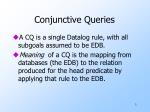Conjunctive Queries
• A CQ is a single Datalog rule, with all subgoals assumed to be EDB.
• Meaning of a CQ is the mapping from databases (the EDB) to the relation produced for the head predicate by applying that rule to the EDB.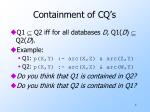Containment of CQ’s
• Q1  Q2 iff for all databases D, Q1(D)  Q2(D).
• Example:
• Q1: p(X,Y) :- arc(X,Z) & arc(Z,Y)
• Q2: p(X,Y) :- arc(X,Z) & arc(W,Y)
• Do you think that Q1 is contained in Q2?
• Do you think that Q2 is contained in Q1?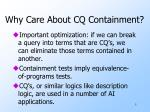Why Care About CQ Containment?
• Important optimization: if we can break a query into terms that are CQ’s, we can eliminate those terms contained in another.
• Containment tests imply equivalence-of-programs tests.
• CQ’s, or similar logics like description logic, are used in a number of AI applications.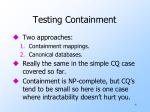Testing Containment
• Two approaches:
• Containment mappings.
• Canonical databases.
• Really the same in the simple CQ case covered so far.
• Containment is NP-complete, but CQ’s tend to be small so here is one case where intractability doesn’t hurt you.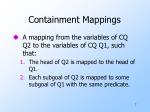Containment Mappings
• A mapping from the variables of CQ Q2 to the variables of CQ Q1, such that:
• The head of Q2 is mapped to the head of Q1.
• Each subgoal of Q2 is mapped to some subgoal of Q1 with the same predicate.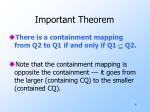Important Theorem
• There is a containment mapping from Q2 to Q1 if and only if Q1  Q2.
• Note that the containment mapping is opposite the containment --- it goes from the larger (containing CQ) to the smaller (contained CQ).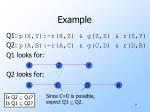Since C=D is possible,

expect Q1  Q2.

Example

Q1: p(X,Y):-r(X,Z) & g(Z,Z) & r(Z,Y)

Q2: p(A,B):-r(A,C) & g(C,D) & r(D,B)

Q1 looks for:

Q2 looks for:

X

Z

Y

A

C

D

B

Is Q2  Q1?

Is Q1  Q2?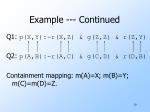Example --- Continued

Q1: p(X,Y):-r(X,Z) & g(Z,Z) & r(Z,Y)

Q2: p(A,B):-r(A,C) & g(C,D) & r(D,B)

Containment mapping: m(A)=X; m(B)=Y; m(C)=m(D)=Z.Example ---Concluded

Q1: p(X,Y):-r(X,Z) & g(Z,Z) & r(Z,Y)

Q2: p(A,B):-r(A,C) & g(C,D) & r(D,B)

• No containment mapping from Q1 to Q2.
• g(Z,Z) can only be mapped to g(C,D).
• No other g subgoals in Q2.
• But then Z must map to both C and D --- impossible.
• Thus, Q1 properly contained in Q2.X

Y

Z

Another Example

Q1: p(X,Y):-r(X,Y) & g(Y,Z)

Q2: p(A,B):-r(A,B) & r(A,C)

Q1 looks for:

Q2 looks for:

Is Q2  Q1?

Is Q1  Q2?

A

B

CExample --- Continued

Q1: p(X,Y):-r(X,Y) & g(Y,Z)

Q2: p(A,B):-r(A,B) & r(A,C)

• Can you find a containment mapping from Q2 to Q1? From Q2 to Q1?
• Is every subgoal in Q1 in the target?
• Can different atoms map to the same atom?Proof of Containment-Mapping Theorem --- (1)
• First, assume there is a CM m : Q2->Q1.
• Let D be any database; we must show that Q1(D)  Q2(D).
• Suppose t is a tuple in Q1(D); we must show t is also in Q2(D).Proof --- (2)
• Since t is in Q1(D), there is a substitution s from the variables of Q1 to values that:
• Makes every subgoal of Q1 a fact in D.
• More precisely, if p(X,Y,…) is a subgoal, then [s(X),s(Y),…] is a tuple in the relation for p.
• Turns the head of Q1 into t.sm maps

each sub-

goal of Q2

to a tuple

of D.

Proof --- (3)
• Consider the effect of applying m and then s to Q2.

head of Q2 :- subgoal of Q2

m m

head of Q1 :- subgoal of Q1

s s

t tuple of D

And the head of Q2 becomes

t, proving t is also in Q2(D); i.e., Q1  Q2.Proof of Converse --- (1)
• Now, we must assume Q1  Q2, and show there is a containment mapping from Q2 to Q1.
• Key idea --- frozen CQ Q :
• For each variable of Q, create a corresponding, unique constant.
• Frozen Q is a DB with one tuple formed from each subgoal of Q, with constants in place of variables.Example: Frozen CQ

p(X,Y):-r(X,Z) & g(Z,Z) & r(Z,Y)

• Let’s use lower-case letters as constants corresponding to variables.
• Then frozen CQ is:

Relation R for predicate r = {(x,z), (z,y)}.

Relation G for predicate g = {(z,z)}.Converse --- (2)
• Suppose Q1  Q2, and let D be the frozen Q1.
• Claim: Q1(D) contains the frozen head of Q1 --- that is, the head of Q1 with variables replaced by their corresponding constants.
• Proof: the “freeze” substitution makes all subgoals in D, and makes the head become the frozen head.Converse --- (3)
• Since Q1  Q2, the frozen head of Q1 must also be in Q2(D).
• Thus, there is a mapping s from variables of Q2 to D that turns subgoals of Q2 into tuples of D and turns the head of Q2 into the frozen head of Q1.
• But tuples of D are frozen subgoals of Q1, so s followed by “unfreeze” is a containment mapping from Q2 to Q1.s followed by inverse of freeze maps each

subgoal p(Y,Z) of Q2 to a subgoal p(A,B) of

Q1 and maps h(X,Y) to h(U,V).

In Pictures

Q2: h(X,Y) :- … p(Y,Z) …

s s

h(u,v) p(a,b) D

freeze

Q1: h(U,V) :- … p(A,B) …Dual View of CM’s
• Instead of thinking of a CM as a mapping on variables, think of a CM as a mapping from atoms to atoms.
• Required conditions:
• The head must map to the head.
• Each subgoal maps to a subgoal.
• As a consequence, no variable is mapped to two different variables.Canonical Databases
• General idea: test Q1  Q2 by checking that Q1(D1)  Q2(D1),…, Q1(Dn)  Q2(Dn), where D1,…,Dn are the canonical databases.
• For the standard CQ case, we only need one canonical DB --- the frozen Q1.
• But in more general forms of queries, larger sets of canonical DB’s are needed.
• Prove that the canonical DB test works (HW)Constants
• CQ’s are often allowed to have constants in subgoals.
• Corresponds to selection in relational algebra.
• CM’s and CM test are the same, but:
• A variable can map to one variable or one constant.
• A constant can only map to itself.CM from Q2 -> Q1 maps X->A and Y->10.

Thus, Q1  Q2.

A CM from Q1 -> Q2 would have to map

constant 10 to variable Y; hence no such

mapping exists.

Example

Q2: p(X) :- e(X,Y)

Q1: p(A) :- e(A,10)Complexity of Containment
• Containment of CQ’s is NP-complete.
• What about determining whether Q1  Q2, when Q1 has no two subgoals with the same predicate?
• What about determining whether Q1  Q2, when Q1 has no three subgoals with the same predicate?
• Sariaya’s algorithm is a linear-time test